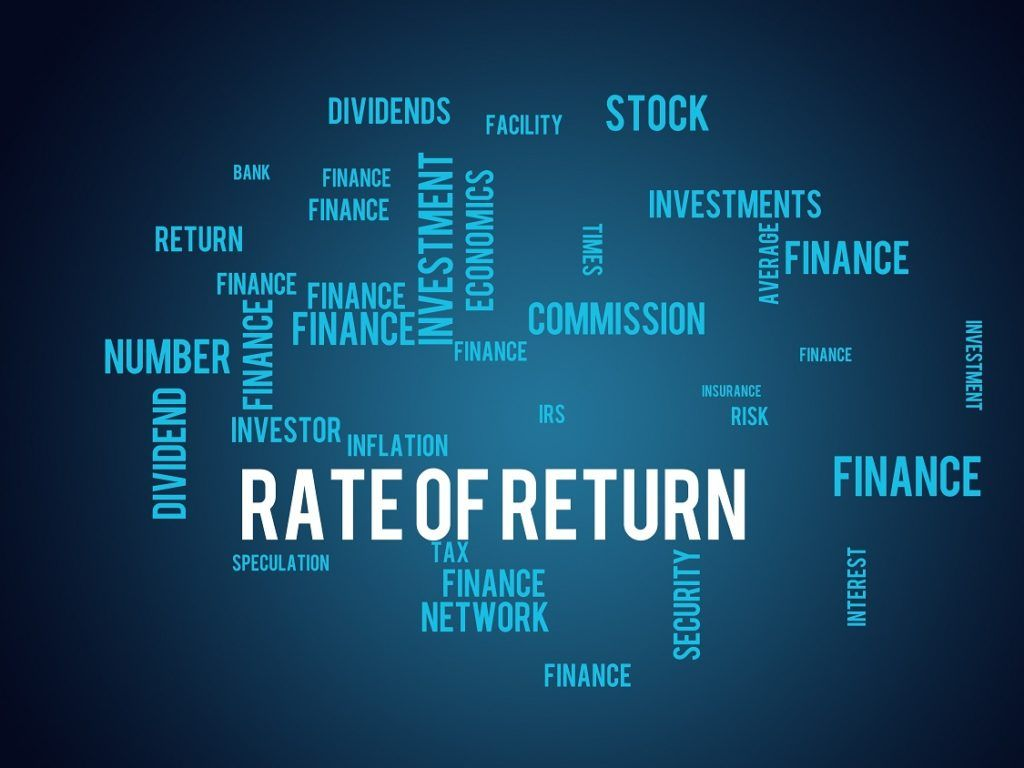# Required Rate of Return

The minimum return that an investor is expecting to receive for their investment

## What is the Required Rate of Return?

The required rate of return (hurdle rate) is the minimum return that an investor is expecting to receive for their investment. Essentially, the required rate is the minimum acceptable compensation for the investment’s level of risk.The required rate of return is a key concept in corporate finance and equity valuation. For instance, in equity valuation, it is commonly used as a discount rate to determine the present value of cash flows.

### Required Rate of Return in Investing

The required rate is commonly used as a threshold that separates feasible and unfeasible investment opportunities. The general rule is that if an investment’s return is less than the required rate, the investment should be rejected.

The metric can be adjusted for the needs and goals of a particular investor. It can consider specific investment goals, as well as risk and inflation expectations.

### How to Calculate the Required Rate of Return?

There are different methods of calculating a required rate of return based on the application of the metric.

One of the most widely used methods of calculating the required rate is the Capital Asset Pricing Model (CAPM). Under the CAPM, the rate is determined using the following formula:

#### RRR = rf + ß(rm – rf)

Where:

• RRR – required rate of return
• rf – risk-free rate
• ß – beta coefficient of an investment
• r– return of a market

The CAPM framework adjusts the required rate of return for an investment’s level of risk (measured by the beta) and inflation (assuming that the risk-free rate is adjusted for the inflation level).

Another method of calculating the required rate is the Weighted Average Cost of Capital (WACC). The WACC approach is frequently utilized in corporate finance. Unlike the CAPM, the WACC takes into consideration the capital structure of a company. Due to this, the required rate obtained from the WACC is used in the corporate decision-making process of undertaking new projects. It can be calculated using the following formula:

#### RRR = wDrD(1 – t) + were

Where:

• w– weight of debt
• r– cost of debt
• t – corporate tax rate
• w– weight of equity
• r– cost of equity

The WACC determines the overall cost of the company’s financing. Therefore, the WACC can be viewed as a break-even return that determines the profitability of a project or an investment decision.

CFI is the official provider of the Financial Modeling and Valuation Analyst (FMVA)™ certification program, designed to transform anyone into a world-class financial analyst.

To keep learning and developing your knowledge of financial analysis, we highly recommend the additional resources below:

• Investing: A Beginner’s Guide
• Discount Factor